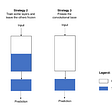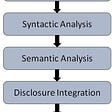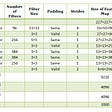# Extended Euclidean Algorithm — Number Theory

Written for publication on samxsmith.com

The Extended Euclidean Algorithm is, as you might imagine, an extension of the standard Euclidean Algorithm. The standard version was developed in order to find the greatest common divisor (GCD) of two numbers. It also allows us to prove Lagrange’s four-square theorem.

The Extended algorithm adds the capability to find the Bézout coefficients for the two input numbers. We’ll cover the Extended algorithm & the Bézout identity but first we need to understand the core algorithm, for finding the GCD of 2 numbers.

# The Euclidean Algorithm

Define `a` & `b` as the two numbers for which we want to find the GCD. Here’s how the algorithm works:

Step 1) If `a` is 0 then `b` is the GCD.

Step 2) Else, set `a = b % a`. and set `b = previous a`. Repeat these steps until `a` is 0. `b` is your GCD.

Here’s an example for finding the GCD of 273 & 399:

`> a = 273, b = 399> a is not 0a₁ = 126 (399 % 273)b₁ = a = 273> a₁ is not 0a₂ = 21 (273 % 126)b₂ = a₁ = 126> a₂ is not 0a₃ = 0 (126 % 21)b₃ = a₂ = 21> a₃ is 0> b₃ is 21> So GCD is 21`

And we can verify that 21 is a divisor of both 462 & 1071:

`273 % 21 = 0399 % 21 = 0`

Why does that work? We can visualise the reasoning using a rectangle, where its lengths take the size of the two numbers in question.

To find a number which divides both 399 and 273, we need to find a square of some size which can tile the entire rectangle. To find the GCD, we are looking for the largest square that can do this.

Euclid’s algorithm works as follows. Take the length of the smaller side of the rectangle. `273` in this case. Fill the rectangle with as many `273 x 273` squares as will fit. Find the sides of the remaining rectangle, if any. One side will have length `273`, the shorter length of the original rectangle.

We filled the rectangle with squares of length `273`, physically dividing it. The remainder of the length is therefore found with `long_side % short_side` e.g. `399 % 273 = 126`. If the remainder is `0`, the whole rectangle is filled. The size of the square is our GCD. Otherwise, we treat the leftover area as a new rectangle, and repeat the process. This continues until no space is left. At that point, the size of the last square used is the GCD.

This is a visual indication of how Euclid’s Algorithm works. The process of redefining `a` & `b` is equivalent to repeating the process of filling a rectangle as much as possible, then choosing a new size of square, and filling again.

21 is our smallest square length size. With those squares we are able to completely cover the rectangle. Because these `21 x 21` squares could cover the whole rectangle we know that 21 literally fits into both `399` & `273`.

That’s how the Euclidean algorithm operates. We can use it to determine what the greatest common divisor of two numbers is, or determine whether two numbers are coprime (have a GCD of 1).

# Bézout’s Identity

Bézout’s Identity states that for the GCD of `a` & `b`, which we’ll call `d`, there are integers `x` & `y` such that `ax + by = d`.

It’s used in modular arithmetic to help find modular multiplicative inverses, because it rearranges like so:

`ax + by = d> subtract d, subtract -byax - d = -by> this tells us that (ax - d) is a multiple of bax - d = (-y)b> So we know thatax % b = d`

More generally, the Bézout Identity gives us a way of reasoning about coprimes in relation to one another. How could we calculate these values? Up steps the Extended Euclidean Algorithm.

# The Extended Euclidean Algorithm

Euclid didn’t stop with GCDs. We can extend the algorithm we looked at earlier to calculate not just the GCD of two numbers, but their Bézout coefficients too. For finding modular multiplicative inverses this algorithm is a one-stop shop.

You’ll remember that Euclid’s algorithm recursively redefined `a` & `b` until we found a divisor which left no remainder. That part of the process does not change. Now though, alongside finding the GCD of `a` & `b` we will also find their Bézout coefficients as we go along.

At each step of the Euclidian Algorithm `b` is the proposed GCD. At the end of the algorithm the final value of `b` is the correct GCD. The Extended Algorithm works with this assumption, by calculating the Bézout coeffecients for each attempt at a GCD, the value `bᵢ`, at each step.

We know the formula for Bézout’s Identity is `ax + by = GCD`. At each step `bᵢ` is the proposed GCD. So `aᵢxᵢ + bᵢyᵢ = bᵢ`. At the beginning this is simple. `b₀` is the proposed GCD.

`> Where i = 0, we want to satisfya₀x₀ + b₀y₀ = b₀> Solved by x₀ = 0, y₀ = 1a₀(0) + b₀(1)= b₀(1) = b₀`

So we have our starting values `x₀, y₀ = 0, 1`. In the following steps the value of `b` will change. We need to adjust `x` & `y` accordingly, so that:

`> for every step, i:a₀xᵢ + b₀yᵢ = bᵢ`

For each new value of the potential GCD, `bᵢ`, we need to find `xᵢ` and `yᵢ` so that they conform to the Bezout Identity for the original `a` and `b` values, `a₀` & `b₀` . This would be intensive, if we did not have the `x` & `y` values from the previous step. With those values we are able to deduce the new ones.

`> Remember how we find b for the next step?bᵢ₊₁ = aᵢ> And also how we find b for the next stepaᵢ = bᵢ₋₁ % aᵢ₋₁> We can substitute "a" out altogetherbᵢ₊₁ = aᵢbᵢ₊₁ = bᵢ₋₁ % bᵢ`

Note: A “Euclidean division” separates the “quotient”, which is the integer part of the result, and the remainder For example `62/7` would give a quotient of `8` and a remainder of `6`. A modulus operation is simply the dividend, `62`, minus the divisor multiplied by the quotient: `7 * 8`. `62 - (7 * 8) = 6`

Another example:

`43 % 8 = 3> ORQUOTIENT OF 43/8 = 543 - (8 * 5) = 3`

With this in mind, let’s start to prep for the Extended algorithm.

`> We want an easy way to find the Bézout Coefficients at each stepbᵢ₊₁ = a₀xᵢ₊₁ + b₀yᵢ₊₁> We know how to find bᵢ₊₁:bᵢ₊₁ = bᵢ₋₁ % bᵢ> Or in terms of Euclidean divisionbᵢ₊₁ = bᵢ₋₁ - bᵢ(QUOTIENT(bᵢ₋₁ / bᵢ))> Let us define the quotient at each step:qᵢ = QUOTIENT(bᵢ₋₁ / bᵢ)> So to find the new b value:bᵢ₊₁ = bᵢ₋₁ - bᵢqᵢ> We will have Bézout's Identity for the previous stepbᵢ₋₁ = a₀xᵢ₋₁ + b₀yᵢ₋₁> And we know the formula for the ith stepbᵢ = a₀xᵢ + b₀yᵢ`

If that all makes sense, now we’re going to do some substitutions using what we’ve established, in order to find some way of actually calculating these Bézout coefficients going forward.

`> given the equations we have derivedbᵢ = a₀xᵢ + b₀yᵢbᵢ₋₁ = a₀xᵢ₋₁ + b₀yᵢ₋₁> and our formula for finding the next b valuebᵢ₊₁ = bᵢ₋₁ - bᵢqᵢ> we can substitute them inbᵢ₊₁ = (a₀xᵢ₋₁ + b₀yᵢ₋₁) - (a₀xᵢ + b₀yᵢ)qᵢbᵢ₊₁ = a₀xᵢ₋₁ + b₀yᵢ₋₁ - a₀xᵢqᵢ - b₀yᵢqᵢ> group a & b termsbᵢ₊₁ = (a₀xᵢ₋₁ - a₀xᵢqᵢ) + (b₀yᵢ₋₁ - b₀yᵢqᵢ)> and factorisebᵢ₊₁ = a₀(xᵢ₋₁ - xᵢqᵢ) + b₀(yᵢ₋₁ - yᵢqᵢ)> compare this to the original Bézout formulabᵢ₊₁ = a₀(xᵢ₊₁) + b₀(yᵢ₊₁)> we can see thatxᵢ₊₁ = xᵢ₋₁ - xᵢqᵢyᵢ₊₁ = yᵢ₋₁ - yᵢqᵢ`

This gives us a way to understand the relationship between the Bézout coefficients at consecutive steps of the Euclidean Algorithm.

Remember these steps from the original algorithm:

Define `a` & `b` as the two numbers for which we want to find the GCD. Here’s how the algorithm works:

Step 1) If `a` is 0 then `b` is the GCD.

Step 2) Else, set `a = b % a`. and set `b = previous a`.

Repeat these steps until `a` is 0. `b` is your GCD.

We need to append to and amend these slightly in order to find both the GCD and Bézout’s Identity.

# Performing the Extended Euclidean Algorithm

Prep Step 1) Define `r₀` & `r₁`. `r₀` is the value `a`, `r₁` is the value `b`. These work just like `a` & `b` but makes successive steps easier to follow.

Prep Step 2) Define `x₀` & `x₁` as `1` & `0` respectively. Also, define `y₀` & `y₁` as `0` & `1` respectively. These values are pre-selected so that Bézout’s Identity is correct from the start. Remember that `r₁` is the initial candidate for GCD. Therefore `r₀x + r₁y = r₁` should be satisfied. This is ensured by setting `y₁ = 1` & `x₁ = 0` because `b = r₁` , therefore `r₀(0) + r₁(1) = r₁` .

Now we have those initial values, we can begin the algorithm.

Step 1) If `rᵢ` is `0`, stop. The GCD value is `rᵢ₋₁`. The Bézout coefficients are `xᵢ₋₁` & `yᵢ₋₁` . This is the base case, which we’ll hit eventually.

Step 2) Find the new quotient, `qᵢ = rᵢ₋₁ / rᵢ`.

Step 3) Find the next value of r, `rᵢ₊₁ = rᵢ₋₁ — (qᵢ * rᵢ)`. (Reminder: this is equivalent to `rᵢ₋₁ % rᵢ`. We use the quotient method because we need the quotient for the following steps.)

Step 4) Find the next value of `x`, `xᵢ₊₁ = xᵢ₋₁ — (qᵢ * xᵢ)`.

Step 5) Find the next value of `y`, `yᵢ₊₁ = yᵢ₋₁ — (qᵢ * yᵢ)`.

That’s how it works. Example time! I’ll include the index, `i`, at the beginning of each cycle to keep track of where we are.

Take the numbers `a = 7` & `b = 93`.

`r₀ = a = 7r₁ = b = 93x₀ = 1x₁ = 0y₀ = 0y₁ = 1> AND begin!i = 1r₁ is not 0q₁ = 0  (quotient of 7/93)r₂ = 7  (7 - 0 * 93)x₂ = 1  (1 - 0 * 0)y₂ = 0  (0 - 0 * 1)i = 2r₂ is not 0q₂ = 13  (quotient of 93 / 7)r₃ = 2   (93 - 13 * 7)x₃ = -13 (0 - 13 * 1)y₃ = 1   (1 - 13 * 0)i = 3r₃ is not 0q₃ = 3  (quotient 7 / 2)r₄ = 1  (7 - 3 * 2)x₄ = 40 (1 - 3 * -13)y₄ = -3 (0 - 3 * 1)i = 4r₄ is not 0q₄ = 2   (quotient 2 / 1)r₅ = 0   (2 - 2 * 1)x₅ = -93 (-13 - 2 * 40)y₅ = 7   (1 - 2 * -3)i = 5r₅ IS 0x = 40  (x₄)y = -3  (y₄)GCD = 1 (r₄)`

There we have it. A greatest common divisor and Bézout coefficients for the values `a = 7`, `b = 93` . A GCD of 1 means they are coprime. We can confirm the Bézout identity is correct by substituting into the equation.

`> ax + by = GCD(7 * 40) + (93 * -3)= 280 - 279= 1`

# That’s It

The Extended Euclidean Algorithm is an important contribution to number theory, very commonly used in cryptography. By finding coefficients for the Bézout Identity and proving whether a number is coprime, it allows us to find modular multiplicative inverses, as used in RSA encryption.

--

--

--

## More from curiositypapers

How the world works. Science, maths & technology applied.

## INTRODUCTION TO NATURAL LANGUAGE PROCESSING## SkyCube — Technical Design and Usecases## ML time series forecasting the right way## Creating a Lane Detection Program Using OpenCV-Python## Human-Activity-Recognition using Transfer Learning and Sequential models## An overview on Natural Language Processing## Dissecting ML Models With NoPdb## Dropout Neural Network Layer In Keras Explained## Sam x Smith

A Software Engineer working in London

## Comprehensive Overview of AlexNet Architecture## Introduction to Cooperative Autonomous Multi-Robot Systems## The Pareto principle — Game-changer to ML Engineers in 2022## Why is superintelligence the last invention humanity needs